# Solution Assignment 01 Hypothesis testing (two or more populations)

1. Younger people cruises
The data for this assignment are listed in the Excel file Younger people cruises.
The cruise ship business is rapidly increasing. Although cruises have long been associated with seniors, it now appears that younger people are choosing a cruise as their vacations. To determine whether this is true, an executive for a cruise line sampled passengers 2 years ago and this year and determined their ages.
a. Formulate the null hypothesis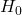and the alternative hypothesis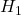.
b. For the inference about comparing the population means of two independent populations (which is the case here) it is important to know whether the population variances are equal. Explain why. A test determines that the population variances may be assumed to be equal. Which test is used and explain why it comes to this conclusion?
c. Do these data allow the executive to infer that cruise ships are attracting younger customers at a 1% significance level? Explain your answer.
d. Estimate with 99% confidence the difference in ages between this year and 2 years ago.

Solution.
a.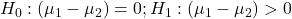b. Applying the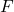-test for the two samples, we find for the test statistic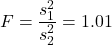. At a 5% significance level, the critical value equals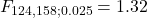. Since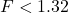we may infer that there is no evidence that the population variances differ. Thus we may apply the equal-variances test.
c. Applying the-test: two-sample assuming equal variances (with the pooled variance estimator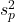) we find for the test statistic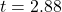.
This is a one-sided test and thus the critical value is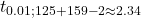. The statistic is in the rejection region and thus there is not enough evidence to accept the null hypothesis. Thus, we may infer at a 1% significance level that cruise ships are attracting younger people. This result is also obtained by using Data Analysis,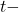test.
d. The 99% confidence interval is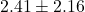.

0